### Iterative Methods: Convergence of Jacobi and Gauss-Seidel Methods

If the matrix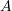is diagonally dominant, i.e., the values in the diagonal components are large enough, then this is a sufficient condition for the two methods to converge. In particular, if every diagonal component satisfies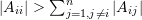, then, the two methods are guaranteed to converge. Generally, when these methods are used, the programmer should first use pivoting (exchanging the rows and/or columns of the matrix) to ensure the largest possible diagonal components. Use the code above and see what happens after 100 iterations for the following system when the initial guess is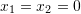: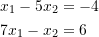The system above can be manipulated to make it a diagonally dominant system. In this case, the columns are interchanged and so the variables order is reversed: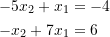This system will now converge for any choice of an initial guess!

To show how the condition on the diagonal componentsis a sufficient condition for the convergence of the iterative methods (solving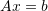), the proof for the aforementioned condition is presented for the Jacobi method as follows. The proof for the Gauss-Seidel method has the same nature.

We want to prove that if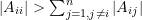, then the Jacobi method (essentially) converges.

To this end, consider the formulation of the Jacobi method, i.e.,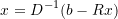Therefore,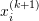, being the approximate solution for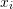at iteration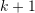, is,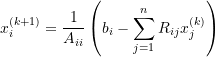Since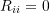(the diagonal components of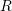are zero), the above equation can be written as,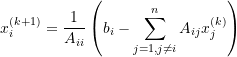Defining the error ofas,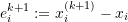we can write,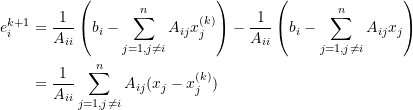which, by the triangular inequality, implies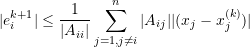Letting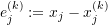, we can write,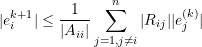where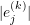is the absolute value of the error of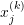(at the k-th iteration). Because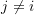, the term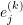does not account for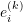being the error of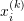.

Now let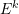be the maximum of the absolute values of the errors of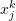for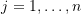; in a mathematical notationis expressed as,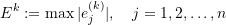Note that, the error of, is also involved in calculating.

Consequently,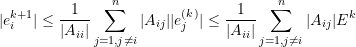or,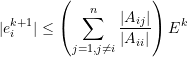This indicates that if the positive value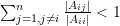, then,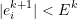which reads the error atiteration is strictly less than the error at k-th iteration. Progressively, the error decreases through the iterations and convergence occurs.

In summary, the “diagonal dominance” conditionwhich can also be written as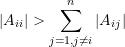is sufficient for the convergence of the Jacobi. This completes the proof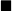.

### Lecture Video

The following video covers the convergence of the Jacobi and Gauss-Seidel Methods.